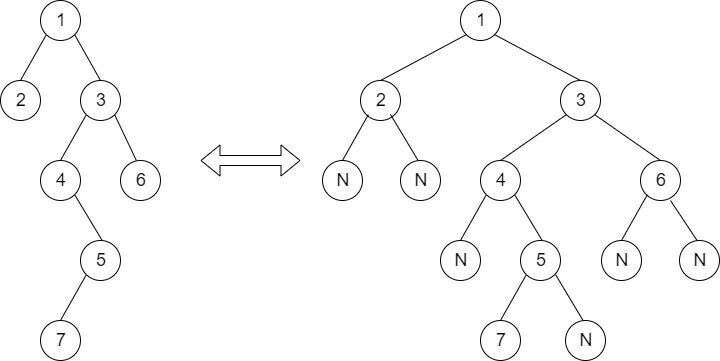Timer is Running

Inorder Successor in BST
##### Submissions: 20260   Accuracy: 39.66%   Difficulty: Easy   Marks: 2
Associated Course(s):   Amazon SDE Test Series

Given a BST,  and a reference to a Node x in the BST. Find the Inorder Successor of the given node in the BST.

Input:
First line of input contains the number of test cases T. For each test case, there will be only two line of input, first line contains a string representing the tree as described below:

1. The values in the string are in the order of level order traversal of the tree where, numbers denotes node values, and a character “N” denotes NULL child.

2. For example:For the above tree, the string will be: 1 2 3 N N 4 6 N 5 N N 7 N

Second line of each test case will contain the integer value K. K is the data of the reference node.

Output:
For each test case output will be the Inorder successor of the given node. If no such successor is present output will be -1.

inOrderSuccessor(). This function takes the root node and the reference node as argument and returns the node that is inOrder successor of the reference node. If there is no successor, return null value.

Expected Time Complexity: O(Height of the BST).
Expected Auxiliary Space: O(1).

Constraints:
1 <= T <= 1000
1 <= N <= 1000, where N is number of nodes
1 <= A[ ] <= 10000

Example:
Input:

2
20 8 22 4 12 N N N N 10 14
8
20 8 22 4 12 N N N N 10 14
10
Output:
10
12

Explanation:
Testcase 1:
20
/      \
8       22
/    \
4     12
/     \
10     14
InOrder traversal gives : 4 8 10 12 14 20 22
Hence successor of 8 is 10

Note: The Input/Ouput format and Example is given are used for the system's internal purpose, and should be used by a user for Expected Output only. As it is a function problem, hence a user should not read any input from the stdin/console. The task is to complete the function specified, and not to write the full code.

#### ** For More Input/Output Examples Use 'Expected Output' option **

Contributor: Harshit Sidhwa,Saksham Raj Seth
Author: Shubham Joshi 1

If you have purchased any course from GeeksforGeeks then please ask your doubt on course discussion forum. You will get quick replies from GFG Moderators there.